# Part 2: Operations with Vectors and Dot Product | Beginner’s Guide to Year 12 Ext 1 Maths

Unsure of vector operations and dot products? Well, you came to the right place!Prepare for your next vector and dot product operations test with this guide! We will discuss vector addition, subtraction, scaling, multiplication and dot products.

## Year 12 Mathematics Extension 1: Operations with Vectors and Dot Product

The topic of Vectors involves both an algebraic and geometric representation of a quantity that includes both a magnitude and direction.

An understanding of vectors enables students to understand how to represent objects in two-dimensional space and is used extensively in many fields of study, including engineering, physics and mathematics.

In this section, we examine the different operations (addition, subtraction and scalar-vector multiplication) of vectors in both algebraic and geometric terms and explore the dot product of two vectors.

## NESA Syllabus Outcomes

Students should be proficient in the following outcome after studies in this topic:

ME-V1.1 Introduction to Vectors

• Perform addition and subtraction of vectors and multiplication of a vector by a scalar algebraically and geometrically, and interpret these operations in geometric terms
• Graphically represent a scalar multiple of a vector (ACMSM012)
• Use the triangle law and the parallelogram law to find the sum and difference of two vectors
• Define and use addition and subtraction of vectors in component form (ACMSM017)
• Define and use multiplication by a scalar of a vector in component form (ACMSM018)

ME-V1.2 Further operations with vectors

• Define, calculate and use the scalar (dot) product of two vectors $$u = x_{1} \ i \ + \ y_{1} \ j$$ and $$v \ = \ x_{2} \ i \ + \ y_{2} \ j$$
• Apply the scalar product,  $$u \cdot v$$ , to vectors expressed in component form, where $$u \cdot v = x_{1}x_{2} + y_{1} y_{2}$$

## Assumed Knowledge

Students should have a basic introductory understanding of vectors and how they are represented.

The process of vector addition must consider both the magnitude and direction of the vectors involved.

There are three methods to add vectors:

• Polygon Rule of Vector Addition

Students are welcomed to utilise the method they prefer as they all yield the same result

Given 2 vectors $$a$$ and $$b$$, we would like to perform $$a + b$$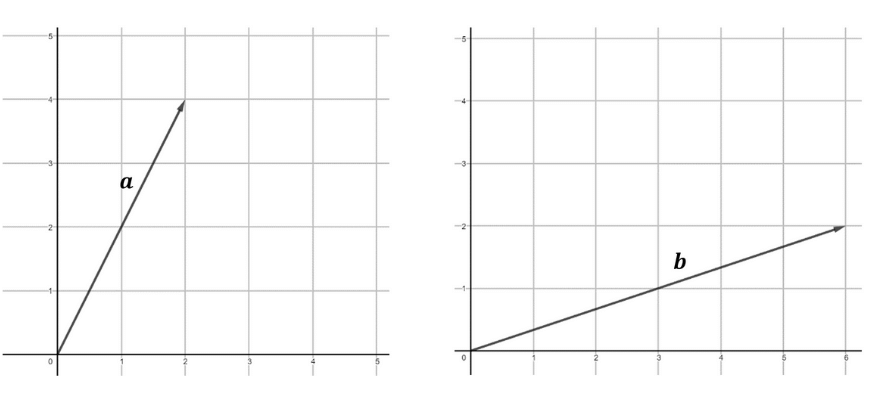Step 1: Sketch vector $$a$$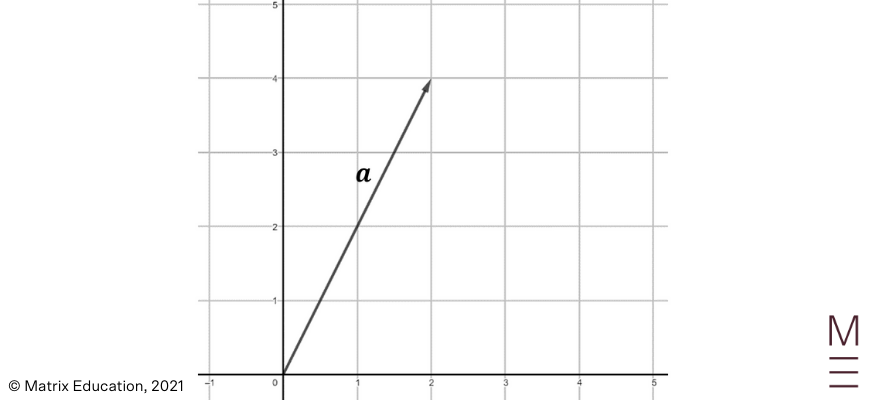Step 2: Position vector $$b$$ such that its tail is on the tip of $$a$$.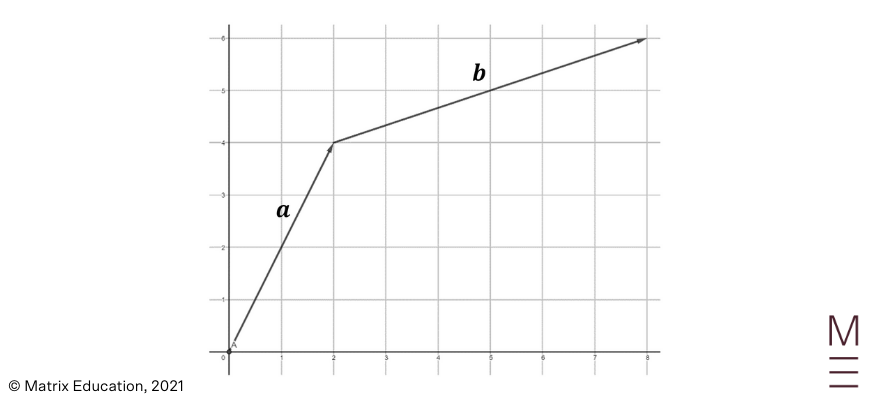Step 3: Connect the tail of vector $$a$$ to the tip of vector $$b$$. This vector is known as the resultant vector and represents the sum $$a + b$$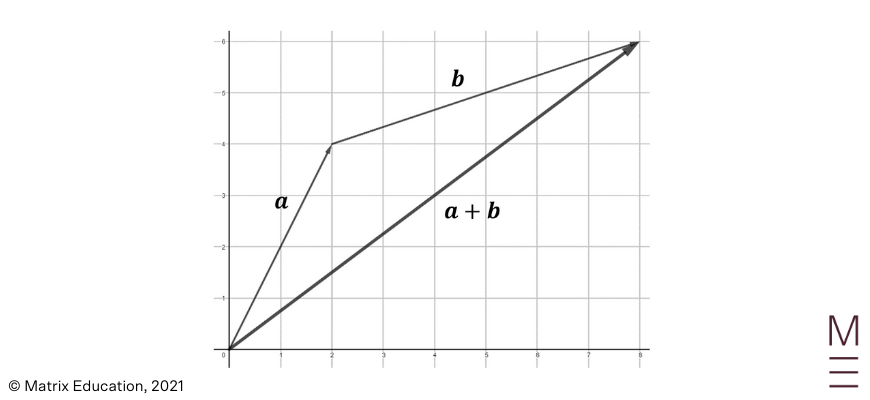When adding two vectors, we can also use a method known as the Parallelogram Law of Addition. This method is essentially the same as tip to tail addition, except now a parallelogram is drawn.

We can also observe from this example that the commutative laws of addition also applies to vectors, such that $$a + b = b+ a$$. This illustrates that the order in which you add the vectors are not important and will product the same resultant vector.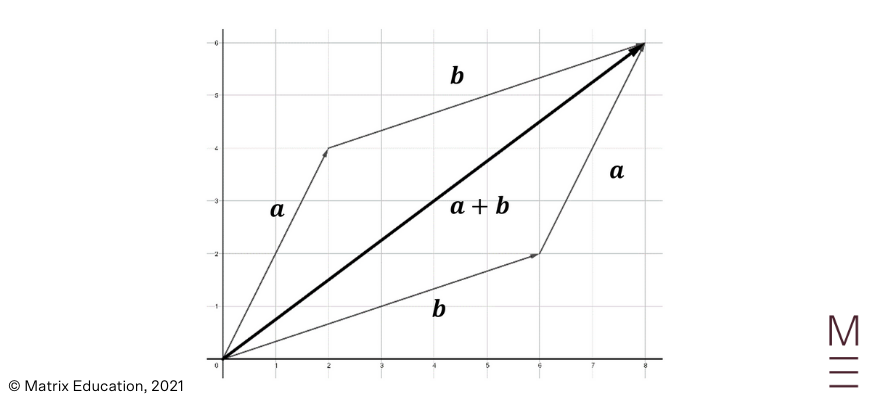### Polygon Rule of Vector Addition

When attempting to add more than two vectors, we can repeatedly use tip to tail addition to produce our resultant vector.

As shown below, the addition may look different depending on the order you sum the vectors in, however, the resultant vector will always be the same.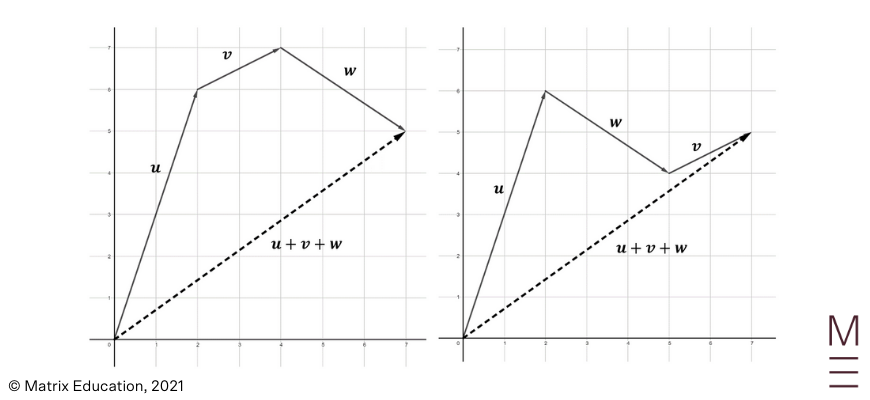## Still rusty with vectors? Practice now!## Vector Subtraction (Geometric)

To subtract a vector, you must add the negative of the vector (using the Tip to Tail method). Mathematically, this can be represented as:

\begin{align*}
u-v = u+(-v)
\end{align*}

Consider the following two vectors $$a$$ and $$b$$.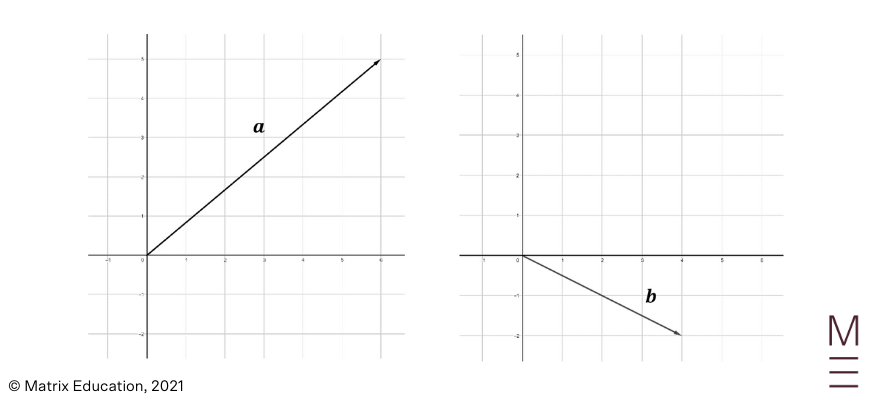To produce $$a-b$$, we must now add vector $$a$$ with vector $$-b$$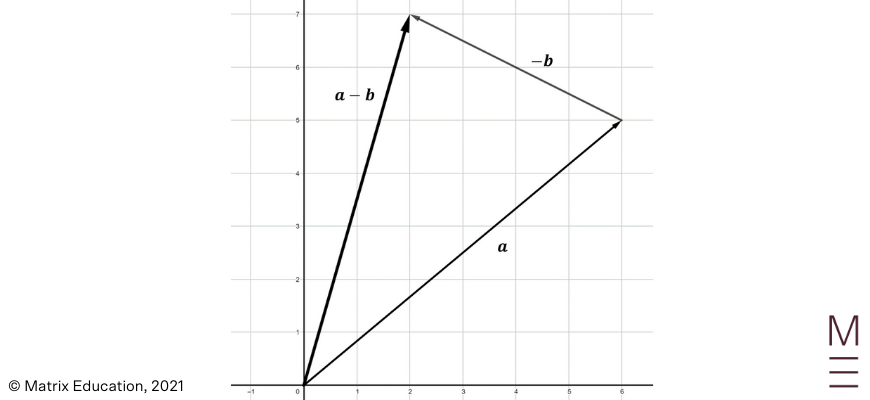## Vector Scaling

Vectors can also be scaled to become shorter, longer or have its direction reversed. A vector $$v$$ can be scaled by a scalar quantity $$\alpha$$ to produce the resultant vector $$\alpha v$$.

A few examples are shown below.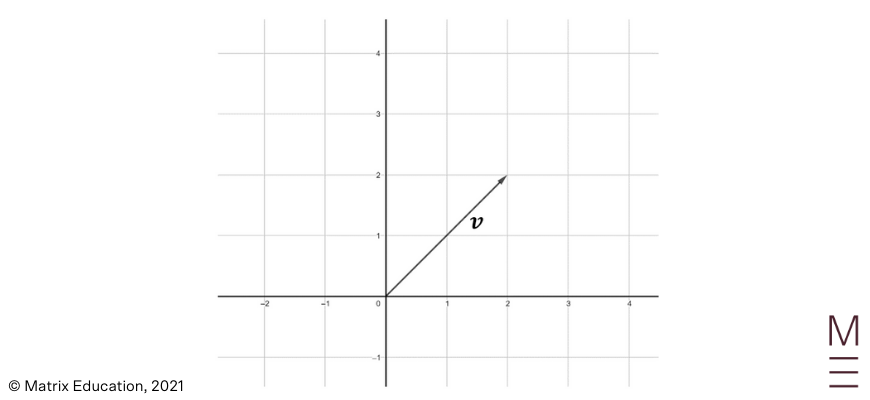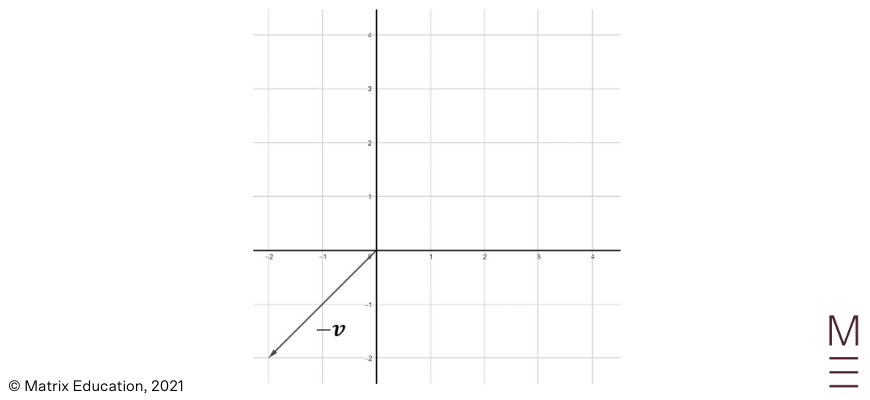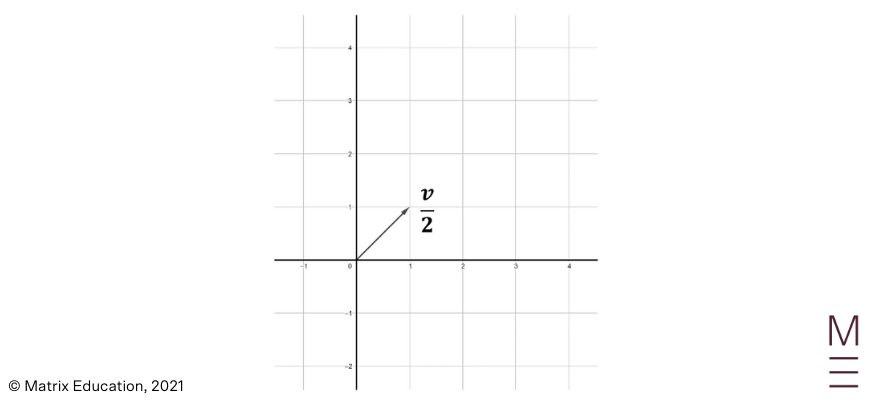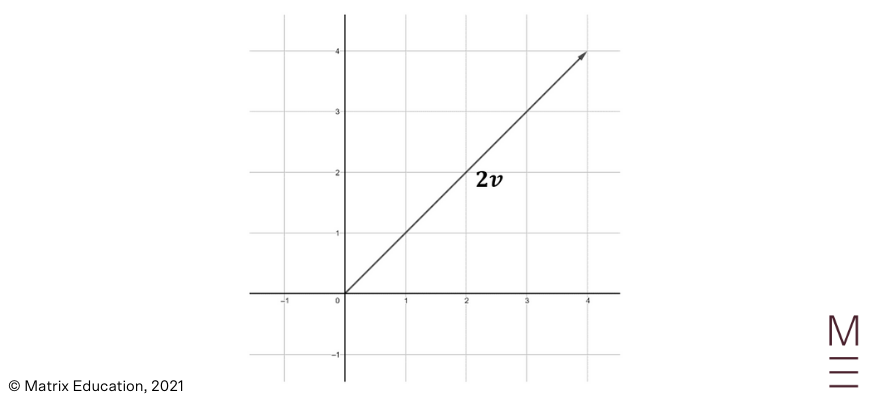## Vector Addition, Subtraction and Scaling (Algebraic)

As you may know, vectors can also be represented algebraically as a column vector as shown below.

Vectors represented in this form is very similar to the coordinate geometry form students are familiar with, with the exception that rather it being a point, it is a vector with the tail placed at the origin.

\begin{align*}
v = \big{(} ^{x}_{y} \big{)}
\end{align*}

Where $$x$$ is the ‘$$x$$ component’ of $$v$$ and $$y$$ is the ‘$$y$$ component’ of $$v$$

Writing out our vectors in algebraic form allows us to easily compute the addition, subtraction and scaling of vectors. For 2 vectors $$u$$ and $$v$$, we can perform vector addition as follows:

\begin{align*}
u = \big{(} ^{a}_{b} \big{)} &, \ \ v = \big{(} ^{c}_{d} \big{)} \\
u + v = \big{(} ^{a}_{b} \big{)} &+ \big{(} ^{c}_{d} \big{)} \ = \ \big{(} ^{a + c}_{b + d} \big{)}
\end{align*}

Likewise, for 2 vectors $$u$$ and $$v$$, we can perform vector subtraction as follows:

\begin{align*}
u = \big{(} ^{a}_{b} \big{)} &, \ \ v = \big{(} ^{c}_{d} \big{)} \\
u – v = \big{(} ^{a}_{b} \big{)} &- \big{(} ^{c}_{d} \big{)} \ = \ \big{(} ^{a – c}_{b – d} \big{)}
\end{align*}

Similarly, for a vector $$v$$, we can scale the vector by a constant $$\alpha$$ as follows:

\begin{align*}
v = \big{(} ^{c}_{d} \big{)} \
\alpha v  = \alpha \big{(} ^{c}_{d} \big{)} = \ \big{(} ^{ \alpha c}_{ \alpha d} \big{)}
\end{align*}

This process is also known as scalar-vector multiplication.

## Vector Dot Product

Previously, we have learnt how to multiply a vector by a scalar, known as scalar-vector multiplication. This section guides you on how to multiply two vectors together, known as the dot product of vectors.

Given two vectors $$u= \big{(} ^{a}_{b} \big{)}$$ and $$v= \big{(} ^{c}_{d} \big{)}$$, the dot product $$u \cdot v$$ is defined as:

\begin{align*}
u \cdot v = ac+bd
\end{align*}

Note: The dot product of two vector produces a scalar number, not a vector.

## Properties of the Dot Product

Let $$u, \ v$$ and $$w$$ be three vectors. Then:

### Rule 1: The dot product is commutative.

It does not matter which vector is ordered first.

\begin{align*}
u \cdot v \ = \ v \cdot u
\end{align*}

### Rule 2: The dot product satisfies the distributive law.

\begin{align*}
u \cdot (v+w) \ = \ v \cdot u + u \cdot w
\end{align*}

### Rule 3: Scalar multiplication is associative.

The order in which you perform the scalar multiplication does not matter.

\begin{align*}
\alpha ( u \cdot v ) \ = \ ( \alpha u ) \cdot v
\end{align*}

### Rule 4: The inner product of a vector with itself results in the square of the magnitude of the vector

\begin{align*}
u \cdot u \ = | u |^{2}
\end{align*}

## Concept Check Questions

1. Consider the following vectors $$a$$ and $$b$$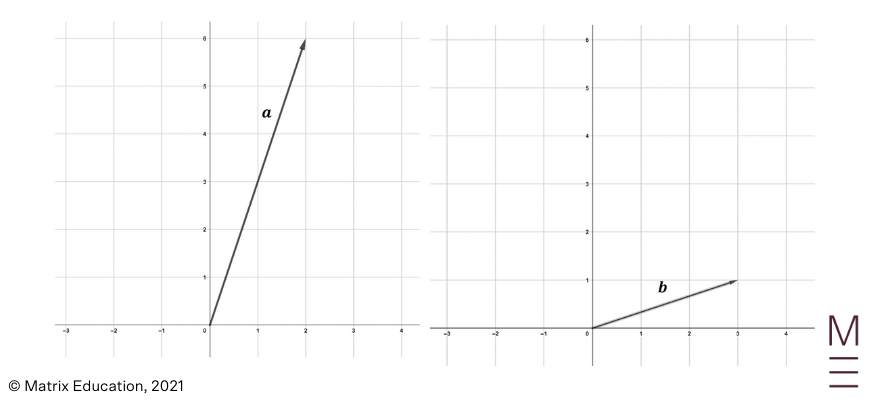Draw each of the following vectors:

a. $$a+b$$

b. $$a-b$$

c. $$-2b+a$$

2. Consider the following vectors

\begin{align*}
a= \big{(} ^{2}_{8} \big{)} , \ \ b= \big{(} ^{-4}_{3} \big{)}, \ \ c= \big{(} ^{5}_{2} \big{)}
\end{align*}

Compute the following:

a. $$a+b$$

b. $$a-c$$

c. $$3a$$

d. $$a+4b-c$$

3. Consider the following vectors

\begin{align*}
a= \big{(} ^{4}_{2} \big{)}, \ \ b= \big{(} ^{-4}_{-2} \big{)}, \ \ c= \big{(} ^{3}_{7} \big{)}
\end{align*}

Compute the following:

a. $$a \cdot b$$

b. $$c \cdot (a+b)$$

c. $$5(a \cdot c)$$

## Concept Check Question Solutions

1.

a.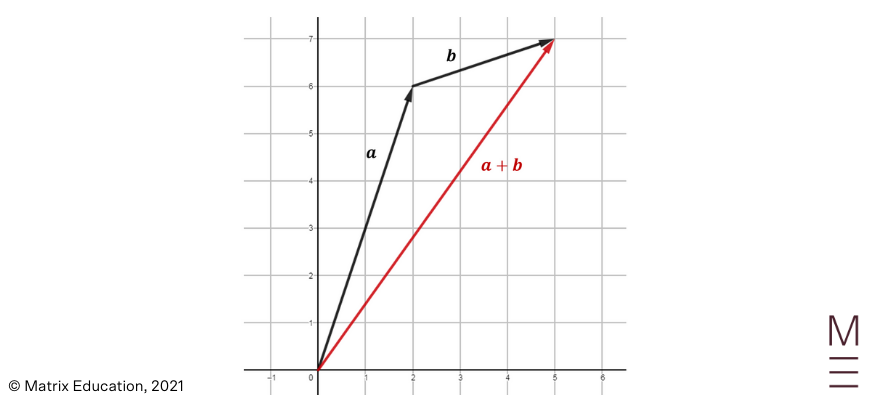b.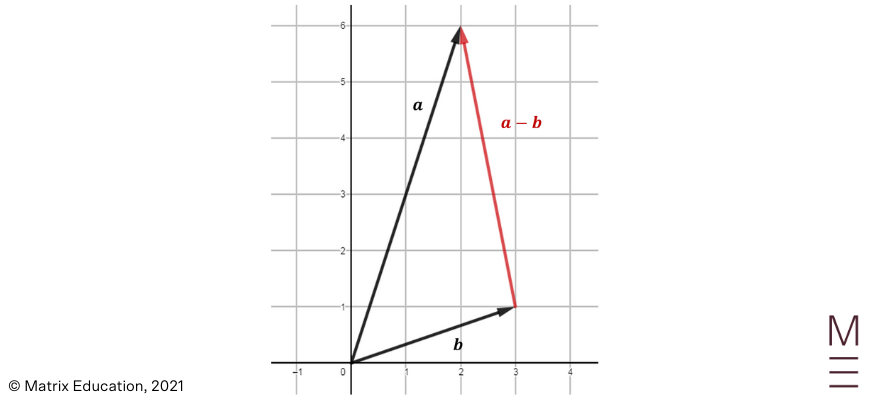c.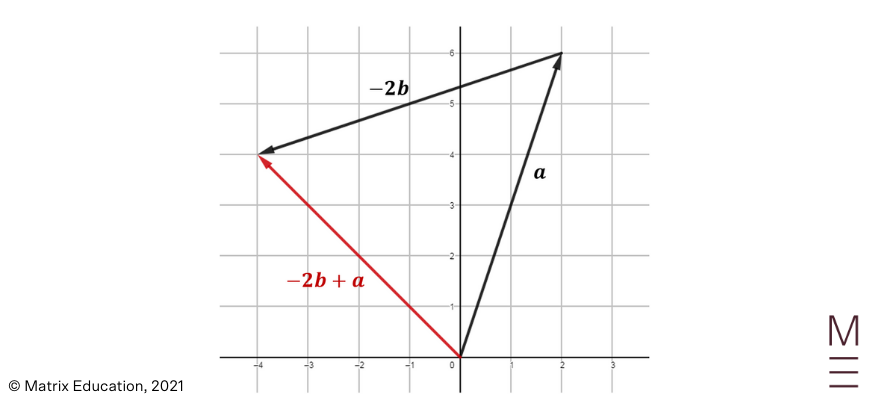2.

a. $$a+b= \big{(} ^{2}_{8} \big{)} + \big{(} ^{-4}_{3} \big{)} = \big{(} ^{-2}_{11} \big{)}$$

b. $$a-c= \big{(} ^{2}_{8} \big{)} – \big{(} ^{5}_{2} \big{)} = \big{(} ^{3}_{6} \big{)}$$

c. $$3a= 3 \big{(} ^{2}_{8} \big{)} = \big{(} ^{6}_{24} \big{)}$$

d. $$a+4b-c = \big{(} ^{2}_{8} \big{)} + 4 \big{(} ^{-4}_{3} \big{)} – \big{(} ^{5}_{2} \big{)} = \big{(} ^{-19}_{18} \big{)}$$

3.

a.

\begin{align*}
a \cdot b &= \big{(} ^{4}_{2} \big{)} \cdot  \big{(} ^{-4}_{-2} \big{)} \\
&= 4 \times -4+2 \times -2 \\
&= -16-4 \\
&= -20
\end{align*}

b.

\begin{align*}
c \cdot (a+b) &= \big{(} ^{3}_{7} \big{)} \cdot \big{(} ^{4}_{2} \big{)} +\big{(} ^{-4}_{-2} \big{)} \\
&=\big{(} ^{3}_{7} \big{)} \cdot \big{(} ^{0}_{0} \big{)} \\
&= 0
\end{align*}

## Ace vector and dot product before your exams!

In our HSC Prep Course, our subject matter experts will break down a year’s worth of Maths content through structured video lessons and provide you with plenty of practice questions and mock exams. Learn more about our HSC Prep Course now.© Matrix Education and www.matrix.edu.au, 2021. Unauthorised use and/or duplication of this material without express and written permission from this site’s author and/or owner is strictly prohibited. Excerpts and links may be used, provided that full and clear credit is given to Matrix Education and www.matrix.edu.au with appropriate and specific direction to the original content.